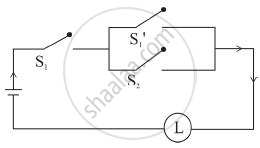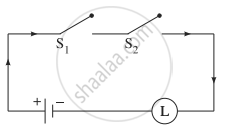# Give an alternative equivalent simple circuit for the following circuit: - Mathematics and Statistics

Sum

Give an alternative equivalent simple circuit for the following circuit:#### Solution

Let p: the switch S1 is closed
q: the switch S2 is closed
∼ p: the switch S1′ is closed or the switch S1 is open
Then the symbolic form of the given circuit is
p ∧ (∼ p ∨ q).
Using the laws of logic, we have,
p ∧ (∼ p ∨ q)
≡ (p ∧ ∼ p) ∨ (p ∧ q) .........(By Distributive Law)
≡ F ∨ (p ∧ q) ...........(By Complement Law)
≡ p ∧ q .........(By Identity Law)
Hence, the alternative equivalent simple circuit is:Concept: Application of Logic to Switching Circuits
Is there an error in this question or solution?